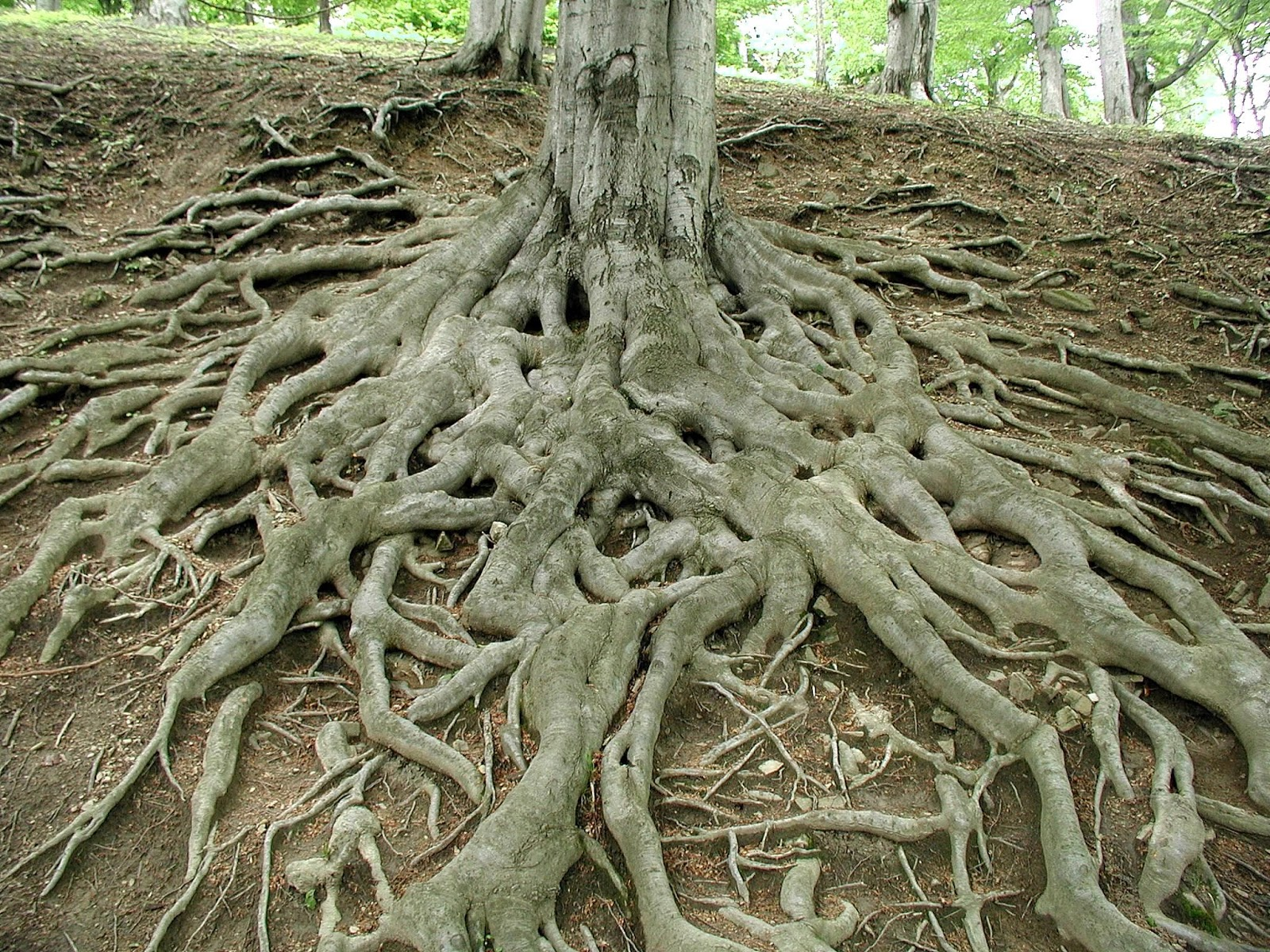# roots

Algebra Level 3r is a root of x^2 + kx = -6 and r+5 is a root of -3x^2 + 3kx = 18

find the value of k

×